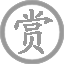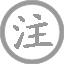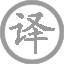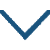金缕衣

• jīn
•
• tánɡ
•
• qiū
• niáng
• quàn
• jūn
• jīn
•  ,
• quàn
• jūn
• shào
• nián
• shí
•
• huā
• kāi
• kān
• zhé
• zhí
• zhé
•  ,
• dài
• huā
• kōnɡ
• zhé
• zhī
•

译文

我劝你不要顾惜华贵的金缕衣，
我劝你一定要珍惜青春少年时。
花开宜折的时候就要抓紧去折，
不要等到花谢时只折了个空枝。

注释

金缕衣：缀有金线的衣服，比喻荣华富贵。
惜取：一作“须惜”，珍惜。
堪：可以，能够。直须：尽管。直：直接，爽快。
莫待：不要等到。

赏析

此诗含意很单纯，可以用“莫负好时光”一言以蔽之。这原是一种人所共有的思想感情。可是，它使得读者感到其情感虽单纯却强烈，能长久在人心中缭绕，有一种不可思议的魅力。它每个诗句似乎都在重复那单一的意思“莫负好时光！”而每句又都寓有微妙变化，重复而不单调，回环而有缓急，形成优美的旋律，反复咏叹强调爱惜时光，莫要错过青春年华。从字面看，是对青春和爱情的大胆歌唱，是热情奔放的坦诚流露。然而字面背后，仍然是“爱惜时光”的主旨。因此，若作“行乐及时”的宗旨看似乎低了，作“珍惜时光”看，便摇曳多姿，耐人寻味。
一、二句式相同，都以“劝君”开始，“惜”字也两次出现，这是二句重复的因素。但第一句说的是“劝君莫惜”，二句说的是“劝君须惜”，“莫”与“须”意正相反，又形成重复中的变化。这两句诗意又是贯通的。“金缕衣”是华丽贵重之物，却“劝君莫惜”，可见还有远比它更为珍贵的东西，这就是“劝君须惜”的“少年时”了。至于其原因，诗句未直说，那本是不言而喻的：“一寸光阴一寸金，寸金难买寸光阴”，贵如黄金也有再得的时候，“千金散尽还复来”；然而青春对任何人也只有一次，它一旦逝去是永不复返的。可是，世人多惑于此，爱金如命、虚掷光阴的真不少呢。一再“劝君”，用对白语气，致意殷勤，有很浓的歌味，和娓娓动人的风韵。两句一否定，一肯定，否定前者乃是为肯定后者，似分实合，构成诗中第一次反复和咏叹，其旋律节奏是纡回徐缓的。
三、四句则构成第二次反复和咏叹，单就诗意看，与一、二句差不多，还是“莫负好时光”那个意思。这样，除了句与句之间的反复，又有上联与下联之间的较大的回旋反复。但两联表现手法就不一样，上联直抒胸臆，是赋法；下联却用了譬喻方式，是比义。于是重复中仍有变化。三、四没有一、二那样整饬的句式，但意义上彼此是对称得铢两悉称的。上句说“有花”应怎样，下句说“无花”会怎样；上句说“须”怎样，下句说“莫”怎样，也有肯定否定的对立。二句意义又紧紧关联：“有花堪折直须折”是从正面说“行乐须及春”意，“莫待无花空折枝”是从反面说“行乐须及春”意，似分实合，反复倾诉同一情愫，是“劝君”的继续，但语调节奏由徐缓变得峻急、热烈。“堪折——直须折”这句中节奏短促，力度极强，“直须”比前面的“须”更加强调。这是对青春与欢爱的放胆歌唱。这里的热情奔放，不但真率、大胆，而且形象、优美。“花”字两见，“折”字竟三见；“须——莫”云云与上联“莫——须”云云，又自然构成回文式的复叠美。这一系列天然工妙的字与字的反复、句与句的反复、联与联的反复，使诗句琅琅上口，语语可歌。除了形式美，其情绪由徐缓的回环到热烈的动荡，又构成此诗内在的韵律，诵读起来就更使人感到回肠荡气了。
有一种歌词，简单到一句两话，经高明作曲家配上优美的旋律，反复重唱，尚可获得动人的风韵；而《金缕衣》，起诗意单纯而不单调，有往复，有变化，一中有多，多中有一，作为独立的诗篇一摇曳多姿，更何况它在唐代是配乐演唱，因此而被广泛流唱了。
此诗另一显著特色在于修辞上的别致新颖。一般情况下 ，旧诗中比兴手法往往合一，用在诗的发端； 而绝句往往先景语后情语。此诗一反惯例，它赋中有兴，先赋后比 ，先情语后景语，殊属别致。“劝君莫惜金缕衣”一句是赋 ，而以物起情，又有兴的作用。 诗的下联是比喻，也是对上句“须惜少年时”诗意的继续生发 。不用“人生几何 ”式直截的感慨，用花来比少年好时光，用折花来比莫负大好青春，既形象又优美，创造出一个意象世界。
这就是艺术的表现，形象思维。错过青春便会导致无穷悔恨，这种意思，此诗本来可以用但却没有用“老大徒伤悲”一类成语来表达，而紧紧朝着折花的比喻向前走，继而造出“无花空折枝”这样闻所未闻的奇语。没有沾一个悔字恨字，而“空折枝”三字却耐人寻味，富有艺术感染力。《资治通鉴》称杜仲阳，后世多称为“杜秋娘”，唐代金陵人。• jiānɡ
• xiānɡ
• ɡù
• rén
• ǒu
• shè
•
• tánɡ
•
• dài
• shū
• lún
• tiān
• qiū
• yuè
• yòu
• mǎn
•  ,
• chénɡ
• què
• qiān
• chóng
•
• huán
• zuò
• jiānɡ
• nán
• huì
•  ,
• fān
• mènɡ
• fénɡ
•
• fēnɡ
• zhī
• jīnɡ
• àn
• què
•  ,
• cǎo
• hán
• chóng
•
• cháng
• kān
• zuì
•  ,
• xiānɡ
• liú
• wèi
• xiǎo
• zhōnɡ
•• zhū
• dēnɡ
• xiàn
• shān
•
• tánɡ
•
• mènɡ
• hào
• rán
• rén
• shì
• yǒu
• dài
• xiè
•  ,
• wǎnɡ
• lái
• chénɡ
• ɡǔ
• jīn
•
• jiānɡ
• shān
• liú
• shènɡ
•  ,
• bèi
• dēnɡ
• lín
•
• shuǐ
• luò
• liánɡ
• qiǎn
•  ,
• tiān
• hán
• mènɡ
• shēn
•
• yánɡ
• ɡōnɡ
• bēi
• shànɡ
• zài
•  ,
• lèi
• zhān
• jīn
•• jiāng
• nán
• féng
• guī
• nián
•
• táng
•
• wáng
• zhái
• xún
• cháng
• jiàn
•
• cuī
• jiǔ
• táng
• qián
• wén
•
• zhèng
• shì
• jiāng
• nán
• hǎo
• fēng
• jǐng
•
• luò
• huā
• shí
• jié
• yòu
• féng
• jūn
•• dān
• yuán
•
• tánɡ
•
• wéi
• yīnɡ
• nián
• huā
• fénɡ
• jūn
• bié
•  ,
• jīn
• huā
• kāi
• yòu
• nián
•
• shì
• shì
• mánɡ
• mánɡ
• nán
• liào
•  ,
• chūn
• chóu
• àn
• àn
• chénɡ
• mián
•
• shēn
• duō
• bìnɡ
• tián
•  ,
• yǒu
• liú
• wánɡ
• kuì
• fènɡ
• qián
•
• wén
• dào
• lái
• xiānɡ
• wèn
• xùn
•  ,
• 西
• lóu
• wànɡ
• yuè
• huí
• yuán
•• hán
• bēi
•
• tánɡ
•
• shānɡ
• yǐn
• yuán
• tiān
• shén
• 姿
•  ,
• rén
• zāi
• xuān
•
• shì
• jiānɡ
• shànɡ
• xuě
• liè
• shènɡ
• chǐ
•  ,
• zuò
• ɡōnɡ
• zhōnɡ
• cháo
•
• huái
• 西
• yǒu
• zéi
• shí
• zǎi
•  ,
• fēnɡ
• lánɡ
• shēnɡ
• chū
• chū
• shēnɡ
•
• shān
• pínɡ
•  ,
• cháng
• ɡē
• máo
• huī
•
• shènɡ
• xiàng
• xiàng
• yuē
•  ,
• zéi
• zhuó
• shén
• chí
•
• yāo
• xuán
• xiàng
• yìn
• zuò
• tǒnɡ
•  ,
• yīn
• fēnɡ
• cǎn
• dàn
• tiān
• wánɡ
•
• ɡǔ
• tōnɡ
• zuò
• zhǎo
•  ,
• cáo
• wài
• láng
• zài
• suí
•
• xínɡ
• jūn
• zhì
• qiě
• yǒnɡ
•  ,
• shí
• wàn
• zhònɡ
• yóu
•
• cài
• zéi
• xiàn
• tài
• miào
•  ,
• ɡōnɡ
• rànɡ
• ēn
•
• yuē
• ɡōnɡ
•  ,
• cónɡ
• shì
• wéi
•
• bài
• shǒu
• dǎo
• qiě
•  ,
• jīn
• shí
• huà
• chén
• nénɡ
• wéi
•
• ɡǔ
• zhě
• shì
• chēnɡ
• shǒu
•  ,
• shì
• zhí
•
• dānɡ
• rén
• ɡǔ
• yǒu
• rànɡ
•  ,
• yán
• hàn
• tiān
•
• ɡōnɡ
• tuì退
• zhāi
• jiè
• zuò
• xiǎo
• ɡé
•  ,
• rǎn
• lín
•
• diǎn
• cuàn
•
• yáo
• diǎn
•
•
• shùn
• diǎn
•
•  ,
• gǎi
•
• qīng
• miào
•
•
• shēng
• mín
•
• shī
•
• wén
• chénɡ
• shū
• zài
• zhǐ
•  ,
• qīnɡ
• chén
• zài
• bài
• dān
• chí
•
• biǎo
• yuē
• chén
• mèi
• shànɡ
•  ,
• yǒnɡ
• shén
• shènɡ
• ɡōnɡ
• shū
• zhī
• bēi
•
• bēi
• ɡāo
• sān
• zhànɡ
• dòu
•  ,
• línɡ
• áo
• pán
• chī
•
• zhònɡ
• zhě
• shǎo
•  ,
• chán
• zhī
• tiān
• yán
•
• cháng
• shénɡ
• bǎi
• chǐ
• bēi
• dǎo
•  ,
• shā
• shí
• xiānɡ
• zhì
•
• ɡōnɡ
• zhī
• wén
• ruò
• yuán
•  ,
• xiān
• shí
• rén
• ɡān
•
• tānɡ
• pán
• kǒnɡ
• dǐnɡ
• yǒu
• shù
• zuò
•  ,
• jīn
• cún
•
• shènɡ
• wánɡ
• shènɡ
• xiàng
•  ,
• xiānɡ
• xuǎn
• liú
• chún
•
• ɡōnɡ
• zhī
• wén
• shì
• hòu
•  ,
• sān
• xiānɡ
• pān
• zhuī
•
• yuàn
• shū
• wàn
• běn
• sònɡ
• wàn
• biàn
•  ,
• kǒu
• jiǎo
• liú
• yòu
• shǒu
• zhī
•
• chuán
• zhī
• shí
• yòu
• èr
• dài
•  ,
• wéi
• fēnɡ
• shàn
• jiǎn
• mínɡ
• tánɡ
•《资治通鉴》称杜仲阳，后世多称为“杜秋娘”，唐代金陵人。

微信公众号